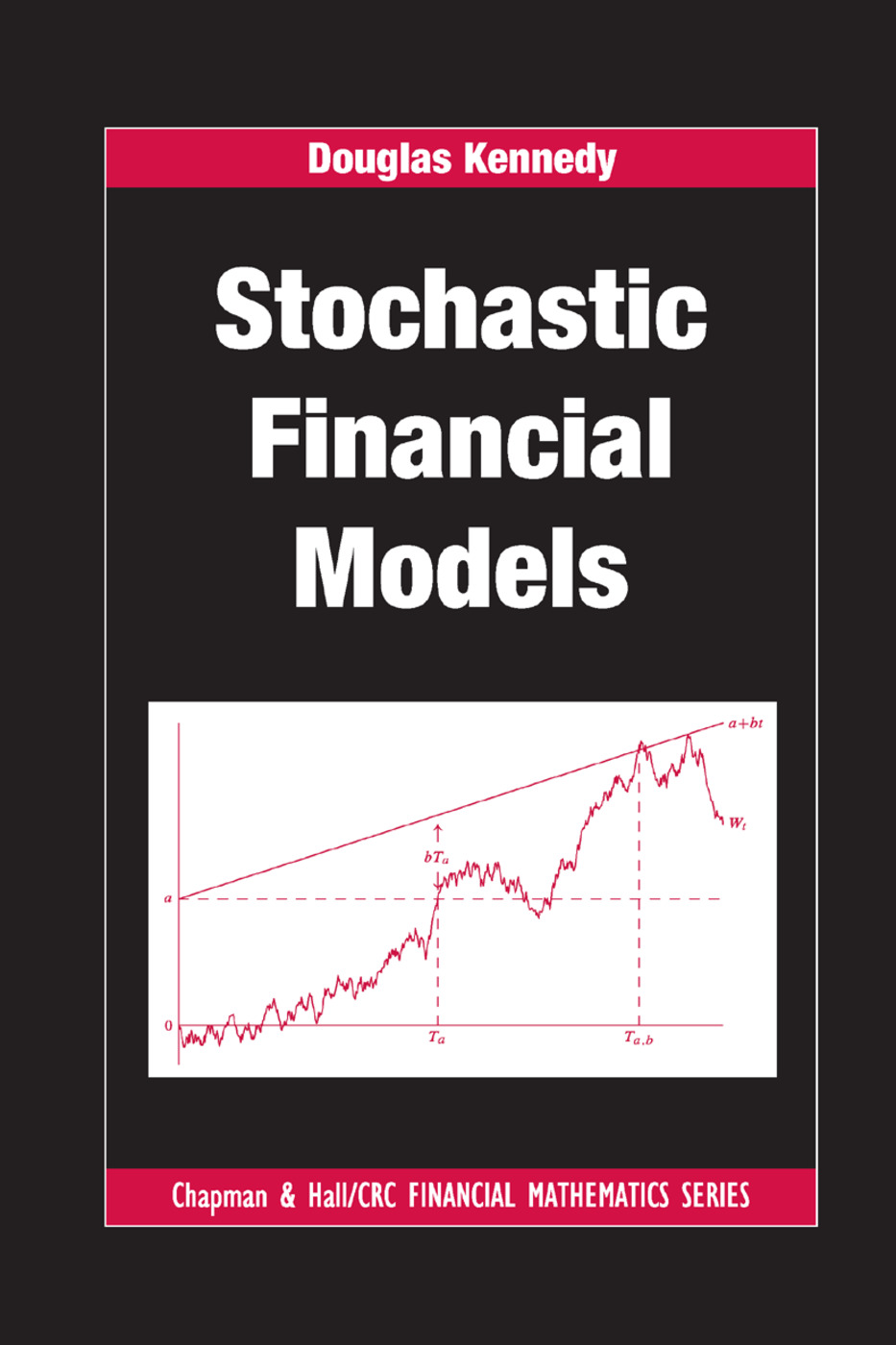Stochastic Financial Models

1st Edition

Chapman and Hall/CRC

264 pages | 26 B/W Illus.

New in Paperback: 9781138381452
pub: 2018-09-10
\$71.00
x
Hardback: 9781420093452
pub: 2010-01-15
\$91.95
x
eBook (VitalSource) : 9780429184789
pub: 2016-04-19
from \$45.98

FREE Standard Shipping!

Description

Filling the void between surveys of the field with relatively light mathematical content and books with a rigorous, formal approach to stochastic integration and probabilistic ideas, Stochastic Financial Models provides a sound introduction to mathematical finance. The author takes a classical applied mathematical approach, focusing on calculations rather than seeking the greatest generality.

Developed from the esteemed author’s advanced undergraduate and graduate courses at the University of Cambridge, the text begins with the classical topics of utility and the mean-variance approach to portfolio choice. The remainder of the book deals with derivative pricing. The author fully explains the binomial model since it is central to understanding the pricing of derivatives by self-financing hedging portfolios. He then discusses the general discrete-time model, Brownian motion and the Black–Scholes model. The book concludes with a look at various interest-rate models. Concepts from measure-theoretic probability and solutions to the end-of-chapter exercises are provided in the appendices.

By exploring the important and exciting application area of mathematical finance, this text encourages students to learn more about probability, martingales and stochastic integration. It shows how mathematical concepts, such as the Black–Scholes and Gaussian random-field models, are used in financial situations.

Reviews

[T]he author covers a number of topics which are normally not addressed in introductions to stochastic finance, and he takes a new and innovative road in the derivation of many familiar results. … the book does contain a lot of interesting material (some of it non-standard) that can enrich a lecture course and deepen the reader’s understanding of financial mathematics, so that it definitely belongs on the shelf of every serious student/teacher in the field. …

—Ruediger Frey, The American Statistician, August 2011

All notions and concepts are defined and well explained. … Specific calculations of any type are included on almost any page and it is clear how important this is for a good understanding of the material. … this well-written book prepared by a well-experienced teacher and researcher will be met with interest by many readers. The wide range of topics discussed in detail makes the book appropriate for courses in financial mathematics at both undergraduate and graduate levels.

Journal of the Royal Statistical Society, Series A, April 2011

This book is a superb beginning level text for senior undergraduate/graduate mathematicians, which is based on lectures delivered by its author to many generations of appreciative Cambridge mathematicians. Many of my own Ph.D. and masters students have taken Dr. Kennedy’s course to uniformly good reviews; this readable book will make its material available to a worldwide audience. I have in the past struggled with some of Dr. Kennedy’s exercises, but the book contains 40 pages of fully worked out solutions to help introduce the reader to the Oxbridge style of learning by problem solving in which even supervisors are sometimes challenged.

—M.A.H. Dempster, Centre for Financial Research, Statistical Laboratory, University of Cambridge, UK

Portfolio Choice

Introduction

Utility

Mean-variance analysis

The Binomial Model

One-period model

Multi-period model

A General Discrete-Time Model

One-period model

Multi-period model

Brownian Motion

Introduction

Hitting-time distributions

Girsanov’s theorem

Brownian motion as a limit

Stochastic calculus

The Black–Scholes Model

Introduction

The Black–Scholes formula

Hedging and the Black–Scholes equation

Path-dependent claims

Dividend-paying assets

Interest-Rate Models

Introduction

Survey of interest-rate models

Gaussian random-field model

Appendix A: Mathematical Preliminaries

Appendix B: Solutions to the Exercises

References

Index

Exercises appear at the end of each chapter.

Douglas Kennedy is a Fellow of Trinity College in Cambridge, UK.